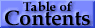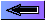### 17.   Spectral Lines: Selection Rules, Intensities, Transition Probabilities, Values, and Line Strengths

Selection rules for discrete transitions
Electric dipole (E1)
("allowed")
Magnetic dipole (M1)
("forbidden")
Electric quadrupole (E2)
("forbidden")

Rigorous rules   1.   Δ J = 0, ± 1
(except 0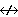0)
Δ J = 0, ± 1
(except 00)
Δ J = 0, ± 1, ± 2
(except 00,
1/21/2, 01)

2. ΔM = 0, ± 1
(except 00
when Δ J = 0)
ΔM = 0, ± 1
(except 00
when Δ J = 0)

ΔM = 0, ± 1, ± 2
3. Parity change No parity change No parity change

With negligible
configuration
interaction
4. One electron
jumping, with
Δl = ± 1,
Δn arbitrary
No change in electron
configuration; i.e., for
all electrons, Δl = 0,
Δn = 0
No change in electron
configuration; or one
electron jumping with
Δl = 0, ± 2, Δn arbitrary

For LS
coupling only
5. ΔS = 0 ΔS = 0 ΔS = 0
6. ΔL = 0, ± 1
(except 00)
ΔL = 0
Δ J = ± 1
ΔL = 0, ± 1, ± 2
(except 00, 01)

• ### Emission Intensities (Transition Probabilities)

The total power ε radiated in a spectral line of frequency ν per unit source volume and per unit solid angle is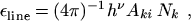(13)

where Aki is the atomic transition probability and Nk the number per unit volume (number density) of excited atoms in the upper (initial) level k. For a homogeneous light source of length l and for the optically thin case, where all radiation escapes, the total emitted line intensity (SI quantity: radiance) is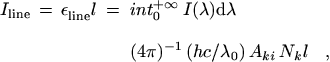(14)

where I(λ) is the specific intensity at wavelength λ, and λ0 the wavelength at line center.

• ## Absorption f values

In absorption, the reduced absorption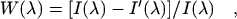(15)

is used, where I(λ) is the incident intensity at wavelength &ambda;, e.g., from a source providing a continuous background, and I′(λ) the intensity after passage through the absorbing medium. The reduced line intensity from a homogeneous and optically thin absorbing medium of length l follows as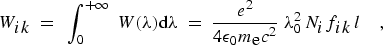(16)
where fik is the atomic (absorption) oscillator strength (dimensionless).

• ### Line Strengths

Aki and fik are the principal atomic quantities related to line intensities. In theoretical work, the line strength S is also widely used (see Atomic, Molecular, & Optical Physics Handbook, Chap. 21, ed. by G.W.F. Drake (AIP, Woodbury, NY, 1996):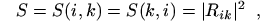(17)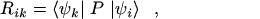(18)

where ψi and ψk are the initial- and final-state wave functions and Rik is the transition matrix element of the appropriate multipole operator P (Rik involves an integration over spatial and spin coordinates of all N electrons of the atom or ion).

• ### Relationships between A, f, and S

The relationships between A, f, and S for electric dipole (E1, or allowed) transitions in SI units (A in s-1, λ in m, S in m2 C2) are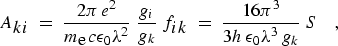(19)

Numerically, in customary units (A in s-1, λ in Å, S in atomic units),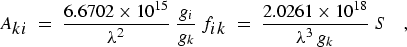(20)

and for S and ΔE in atomic units,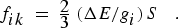(21)

gi and gk are the statistical weights, which are obtained from the appropriate angular momentum quantum numbers. Thus for the lower (upper) level of a spectral line, gi(k) = 2 Ji(k) + 1, and for the lower (upper) term of a multiplet,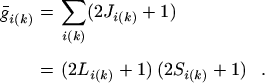(22)

The Aki values for strong lines of selected elements are given. For comprehensive numerical tables of A, f, and S, including forbidden lines (see Sources of Spectroscopic Data).

Experimental and theoretical methods to determine A, f, or S values as well as atomic lifetimes are discussed in Atomic, Molecular, & Optical Physics Handbook, Chaps. 17, 18, and 21, ed. by G.W.F. Drake (AIP, Woodbury, NY, 1996).

Conversion relations between S and Aki for the most common forbidden transitions
SI units a   Numerically, in
customary units b

Electric quadrupole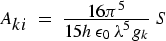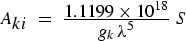Magnetic dipole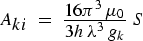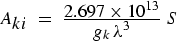a A in s-1, λ in m.  Electric quadrupole: S in m4 C2. Magnetic dipole: S in J2 T-2. b A in s-1, λ in Å. S in atomic units: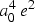= 2.013 × 10-79 m4 C2 (electric quadrupole),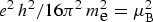= 8.601 × 10-47 J2 T-2 (magnetic dipole). µB is the Bohr magneton.

Oscillator strengths f are not used for forbidden transitions, i.e., magnetic dipole (M1), electric quadrupole (E2), etc.

[Numerical example: For the 1s2p 1P- 1s3d 1D2 (allowed) transition in He I at 6678.15 Å: gi = 3; gk = 5; Aki = 6.38 × 107 s-1; fik = 0.711; S = 46.9 a02 e2.]

• ### Relationships between Line and Multiplet Values

The relations between the total strength and f value of a multiplet (M) and the corresponding quantities for the lines of the multiplet (allowed transitions) are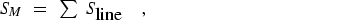(23)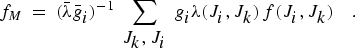(24)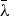is the weighted ("multiplet") wavelength in vacuum: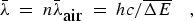(25)
where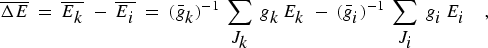(26)
and n is the refractive index of standard air.